# Asg 2: Ray Traced Transmission

## Objectives

To implement transmission rays, rendering transparent objects

## Assignment

1. Using your parallelized ray tracer, rewrite your `ray_t::trace()` routine to spawn a transmission ray for any object intersected that has `alpha > 0`.
2. Transmission rays, unlike reflection rays which bounce off the object surface, cross into the object, refracting as they do so, according to Snell's Law, which states that n1 sin(θ1) = n2 sin(θ2) where n1 is the index of refraction of medium 1 w.r.t. vacuum and n2 is the index of refraction of medium 2 w.r.t. vacuum. An index of refraction, or `ior = 1.000293` models air, while an index of refraction of `1.337` models the vitreous humor—the liquid inside the human eye (see the list of refractive indices for others).
3. The angle of refraction of the transmission ray, assuming incoming normalized direction u (the ray direction), is calcuated as:
```(n1/n2)(u - cosθ1N) - cosθ2N
```
where
• N is the normal at the intersection point (which you get from your `model_t::find_closest()`),
• cosθ1 is u·N,
• cosθ2 is sqrt(1 - ((n1/n2)2(1 - (u·N)2)))
Note that you can set n1 = 1.000293 and never change it if we assume the scene is immersed in air.
4. A transmission ray is spawned in much the same way as a reflection ray, except that it is only done if the intersected object's `alpha` value is `> 0` (in other words only when the ray encounters a transparent object).
5. Take care to refract the ray about a properly signed surface normal, i.e., if u·N < 0 (the incoming ray direction u points into the object) then refract the ray about N with n2 otherwise (the incoming ray direction u points out of the object) refract the ray about -N with 1/n2.
6. Blend the resultant color via the traditional compositing (linear interpolation) equation: ```color = ((1.0 - alpha) * color) + (alpha * transmitted_color); ``` where `transmitted_color` is the transparent color set by the transmission ray (don't forget to clamp the color to [0,1] range).
7. With the `model.txt` given below, your ray tracer should now render the image below, of a transparent sphere with the same refractive index as the human eye's vitreous humor.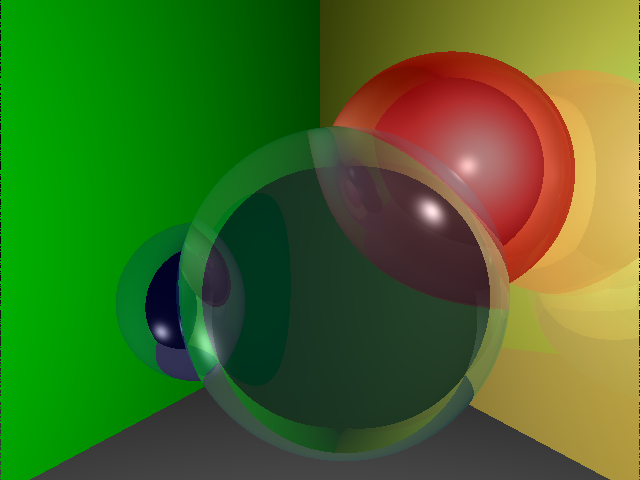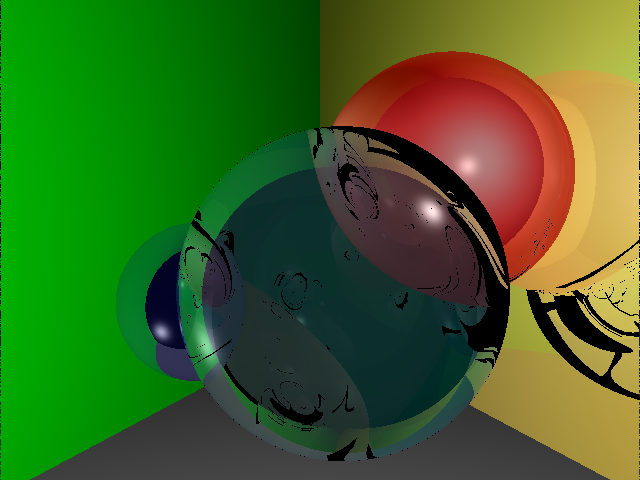no TIR, no t1 t1 but no TIR (oops!) TIR but no t1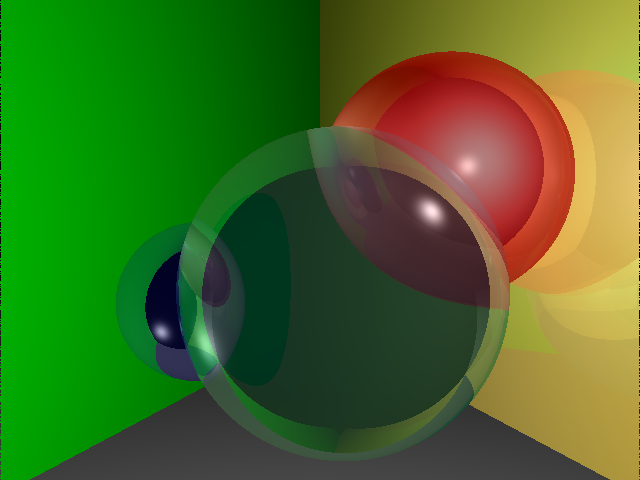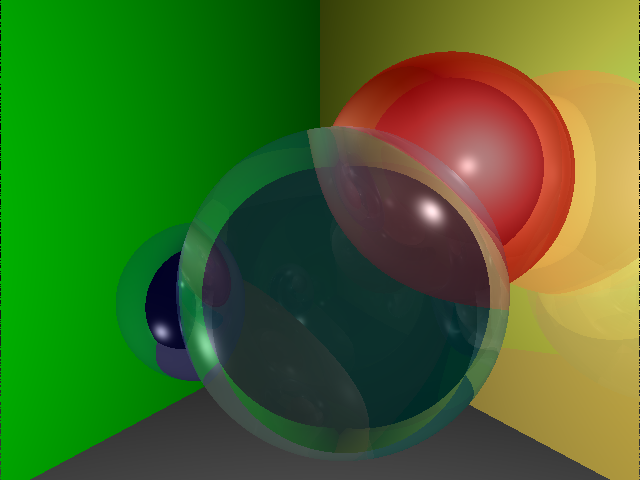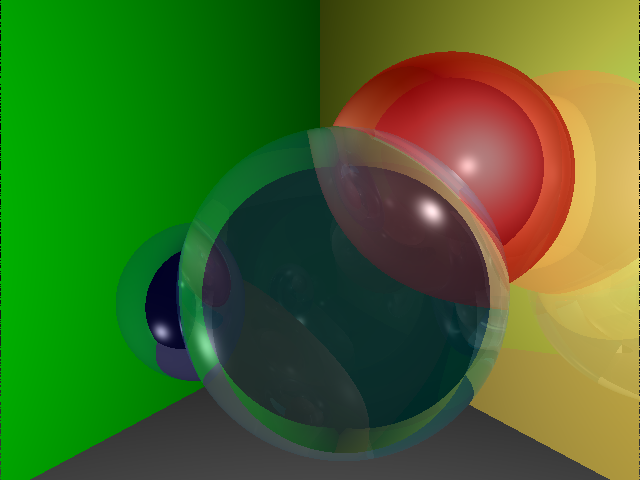no reflection? (doesn't seem right, I forget how I got this, sorry) final image with Schlick's approximation

## Details

1. Rewrite your `material_t` to handle processing material properties `alpha` (a single `float` value) and `ior` (also a single `float` value). Make sure that your `material_t` class provides queries that return these values, e.g., `getalpha()` and `getior()`, which will behave similarly to `getspec()` for example, but will return single `float` values instead of `vec_t` vectors. Before doing anything on this assignment, make sure that you can successfully process the input `model.txt` file and be able to write it out.
2. The old `model_t::find_closest()` routine won't work anymore because its initial design assumed that a ray would hit any given object only once (and reflect). Clearly this won't work for transparent objects that have volume, through which the transparent ray needs to exit, once it has penetrated it. This means, particularly for transparent spheres, that the ray will hit the sphere twice, and so the `find_closest()` routine needs to be rewritten:
1. initialize the local variable `closest_dist` to `INFINITY` instead of to `-1`
2. remove the `obj==last_obj ||` evaluation from the initial call to `obj->hits()`—the current object should only be skipped if the returned `dist < 0`
3. only record the closest distance, object, hit point, and normal if `(0.00001 < dist) && (dist < closest_dist)` (the distance to the intersection point must be non-zero)
3. Because the refracted ray is refracted either about N or -N, it is convenient to write a ``` vec_t::refract(const vec_t& n, double n_t) const ``` function that refracts the given (e.g., `this`) vector about `n` with n2 = nt. Writing a ``` vec_t::reflect(const vec_t& n) const ``` function for reflection is also a good idea, where the resultant reflection vector is u - 2.0(u·N)N.
4. When calculating refraction, if the expression inside the radical is less than 0, i.e., if 1 - ((n1/n2)2(1 - (u·N)2)) < 0 then the refraction angle is critical and the ray does not refract, but reflects internally. This is known as total internal reflection, or TIR. When this happens, simply reflect the ray about N.
5. When a ray enters a transparent sphere, the sphere's `hits` routine should now calculate both roots of the quadratic equation and return the smaller of the two, unless the smaller of the two is less than `0.00001` in which case the larger should be returned. Instead of just calculating `t` now calculate ``` t0 = (-b - sqrt(d))/(2.0*a); t1 = (-b + sqrt(d))/(2.0*a); ``` and return `t0` if it is smaller than `t1` unless it is behind the ray position (i.e., unless `t0` is negative, but due to round-off error we test whether it smaller than `0.00001`).
Note that if `t1` is returned, then we are exiting the sphere.
6. The above calculation of `t1` can in turn lead to infinite loops where rays can get stuck in a region close to the sphere surface. To prevent this, rewrite `ray_t::trace()` to include an additional integer argument, `bounce` which you test immediately as you enter the routine—if it is larger than say 10, return (prune this ray as it has already bounced too many times).

## Optional

1. If you implement both `vec_t::reflect()` and `vec_t::refract()` functions, then you can try implementing Pete Shirley's solution (see §10.7) which uses Schlick's approximation to the Fresnel equations for light transport (both reflection and refraction of light may occur at the surface interface where refractive indices differ). In this case two rays are spawned when intersecting a transparent surface: a reflection and a transmission ray. The result is linearly interpolated via the `R` parameter:
```	R = R0 + (1-R0)(1-cosθ)5
```
Blending is then done by using R as the linear interpolant between the reflected and transmitted colors, then this resultant color is used just as the transmitted color was blended with the surface color above.

## Input

1. The `model.txt` file used to generate the above image is: ```camera cam1 { pixeldim 640 480 worlddim 8 6 viewpoint 4 3 6 } light centerback { location 3 4 -1 emissivity 1 1 1 } light backleft { location 1 1 0 emissivity 1 1 1 } light topright { location 6 4 1 emissivity 1 1 1 } material green { ambient 0 5 0 } material red { ambient 5 0 0 diffuse .7 .7 .7 specular .3 .3 .3 } material blue { ambient 0 0 5 diffuse .2 .2 .2 specular .8 .8 .8 } material cyan { ambient 0 4 5 diffuse .2 .2 .2 specular .8 .8 .8 alpha .4 ior 1.337 } material yellow { ambient 5 4 0 diffuse .7 .7 .7 specular .3 .3 .3 } plane leftwall { material green normal 3 0 1 point 0 0 0 } plane rightwall { material yellow normal -3 0 1 point 8 0 0 } material gray { ambient 2 2 2 } plane floor { material gray normal 0 1 0 point 0 -0.2 0 } sphere upperright { material red center 6 4 -2 radius 2 } sphere central { material blue center 2 2 -2 radius 1.3 } sphere vitreous { material cyan center 4.3 2.3 -1 radius 2.3 } ```

## Turn in

Turn in all of your code, in one `tar.gz` archive of your asg##/ directory, including:
1. A `README` file containing
1. Course id--section no
2. Name
3. Lab description
4. Brief solution description (e.g., program design, description of algorithm, etc., however appropriate).
5. Lessons learned, identified interesting features of your program
6. Any special usage instructions
2. `Makefile`
3. source code (`.h` headers and `.cpp` source)
4. object code (do a `make clean` before `tar`)

## How to hand in

See `handin` notes